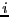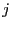Next: Matrix inverse Up: Calculating determinant Previous: Scalar and interval matrix   Contents

Polynomial matrix

A polynomial matrix is assumed is assumed here to be a matrix whose elements are univariate polynomial in the same variable. The determinant of such matrix (which is a polynomial) may be computed with the procedure

int Determinant_Characteristic(POLYNOMIAL_MATRIX A,
INTERVAL_VECTOR &Coeff)
where
• A: a POLYNOMIAL_MATRIX structure which describes the polynomial matrix. This structure is composed of
• dim: the dimension of the matrix
• Coeff: an interval vector with indicates the coefficients of the polynomial in the matrix, row by row
• Order: an integer matrix. m=Order(i,j) indicates that the element at the-th row and-th column has coefficients Coeff(l) to Coeff(m) where l is Coeff(i,j-1)+1 if j is greater than 1, Coeff(i-1,dim) otherwise. Hence Order(1,1)=3 indicate that the first elements has as coefficient Coeff(1), Coeff(2), Coeff(3) and hence is a second order polynomial
• Coeff: the coefficients of the determinant polynomial
Note that this procedure is safe: even for a scalar matrix the coefficients of the determinant will always include the coefficient of the exact determinant.Next: Matrix inverse Up: Calculating determinant Previous: Scalar and interval matrix   Contents
Jean-Pierre Merlet 2012-12-20